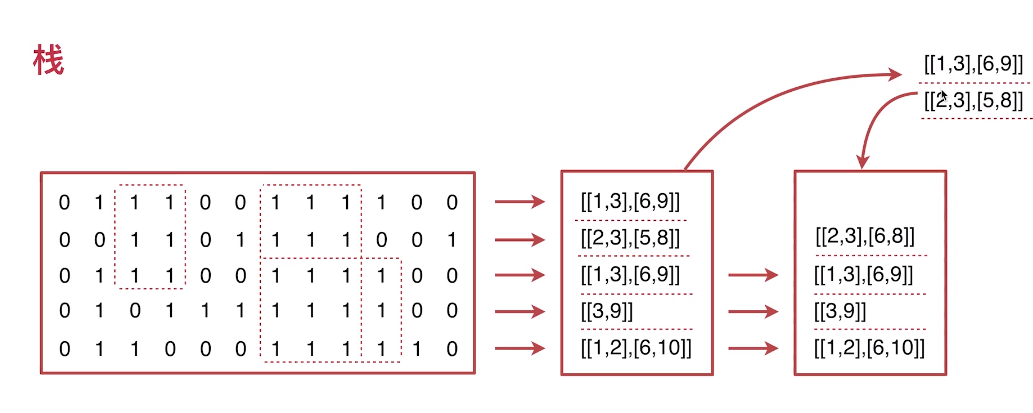# JS基础算法(数据结构之栈篇)

• 前端
• 面试
• 算法原创

### 数据结构之栈

#### 棒球比赛

1.整数（一轮的得分）：直接表示您在本轮中获得的积分数。
2.“+”（一轮的得分）：表示本轮获得的得分是前两轮有效 回合得分的总和。
3.“D”（一轮的得分）：表示本轮获得的得分是前一轮有效 回合得分的两倍。
4.“C”（一个操作，这不是一个回合的分数）：表示您获得的最后一个有效 回合的分数是无效的，应该被移除。

#### 示例 2:

export default (arr)=>{
// 用数组来实现堆栈结构，pop,push(从后面添加，从后面删除)
let result=[]
// 上一轮的数据
let pre1
// 上上轮的数据
let pre2
// 对数组进行遍历，遍历的目的是处理得分
arr,forEach(item=>{
switch(item){
case 'C':
if(result.length){
result.pop()
}
break
case 'D':
pre1=result.pop()
result.push(pre1,pre1*2)
break
case '+':
pre1=result.pop()
pre2=result.pop()
result.push(pre2,pre1,pre1+pre2)
break
default:
result.push(item*1)
break
}
})
return result.reduce((total,num)=>{total+num})
}


#### 最大矩形

##### 示例:

[
[“1”,“0”,“1”,“0”,“0”],
[“1”,“0”,“1”,“1”,“1”],
[“1”,“1”,“1”,“1”,“1”],
[“1”,“0”,“0”,“1”,“0”]
]export default (arr) => {
let result = []
let reg = /1{2,}/g
// 把二位数组重新表达，把相邻的1提取出来（起始点+截止点）
arr = arr.map(item => {
let str = item.join('')
let r = reg.exec(str)
let rs = []
while (r) {
rs.push([r.index, r.index + r.length - 1])
r = reg.exec(str)
}
return rs
})
// 通过递归计算相邻的矩阵
let maxRect = (arr, result, n = 1) => {
// 弹出第一行
let top = arr.pop()
// 弹出第二行
let next = arr.pop()
// 记录第一行的每一个起始点和截止点
let tt
// 记录第二行的每一个起始点和截止点
let nn
// 记录交叉的起始索引
let start
// 记录交叉的截止索引
let end
let width = 1
let maxWidth = 1
n++
for (let i = 0, il = top.length; i < il; i++) {
tt = top[i]
for (let j = 0, jl = next.length; j < jl; j++) {
nn = next[j]
width = Math.min(tt, nn) - Math.max(tt, nn)
// 修改避免相邻两个数的差值为1（实际宽度为2）没有为start,end赋值导致的bug,应该加上=
if (width >= maxWidth) {
maxWidth = width
start = Math.max(tt, nn)
end = Math.min(tt, nn)
}
}
}
// 如果没有找到交叉点
if (start === undefined || end === undefined) {
if (n < 3) {
return false
} else {
width = top - top + 1
if (width > 1) {
result.push((n - 1) * width)
}
}
} else {
// 找到交叉点继续下一行
if (arr.length > 0) {
arr.push([
[start, end]
])
maxRect(arr, result, n++)
} else {
// 从某一行一直计算到最后一行，这个时候start和end一直有值，所以不会进入到if层，这个时候n就是累计的行数（高），end-start+1就是宽
result.push(n * (end - start + 1))
}
}
}
while (arr.length > 1) {
maxRect([].concat(arr), result)
arr.pop()
}
// 取最大值
let max = 0
let item = result.pop()
while (item) {
if (item > max) {
max = item
}
item = result.pop()
}
return max > 0 ? max : -1
}

export default (arr) => {
let result = []
let reg = /1{2,}/g
// 把二位数组重新表达，把相邻的1提取出来（起始点+截止点）
arr = arr.map(item => {
let str = item.join('')
let r = reg.exec(str)
let rs = []
while (r) {
rs.push([r.index, r.index + r.length - 1])
r = reg.exec(str)
}
return rs
})
// [ [], [ [ 2, 4 ] ], [ [ 0, 4 ] ], [] ]
// 通过递归计算相邻的矩阵
let maxRect = (arr, result, n = 1) => {
// 弹出第一行
let top = arr.pop()
// 弹出第二行
let next = arr.pop()
// 记录第一行的每一个起始点和截止点
let tt
// 记录第二行的每一个起始点和截止点
let nn
// 记录交叉的起始索引
let start
// 记录交叉的截止索引
let end
let width = 1
let maxWidth = 1
n++
for (let i = 0, il = top.length; i < il; i++) {
tt = top[i]
for (let j = 0, jl = next.length; j < jl; j++) {
nn = next[j]
width = Math.min(tt, nn) - Math.max(tt, nn)
// 修改避免相邻两个数的差值为1（实际宽度为2）没有为start,end赋值导致的bug,应该加上=
if (width >= maxWidth) {
maxWidth = width
start = Math.max(tt, nn)
end = Math.min(tt, nn)
}
}
}
// 如果没有找到交叉点
if (start === undefined || end === undefined) {
if (n < 3) {
return false
} else {
width = top - top + 1
if (width > 1) {
result.push((n - 1) * width)
}
}
} else {
// 找到交叉点继续下一行
if (arr.length > 0) {
arr.push([
[start, end]
])
maxRect(arr, result, n++)
} else {
// 从某一行一直计算到最后一行，这个时候start和end一直有值，所以不会进入到if层，这个时候n就是累计的行数（高），end-start+1就是宽
result.push(n * (end - start + 1))
}
}
}
while (arr.length > 1) {
maxRect([].concat(arr), result)
arr.pop()
}
// 取最大值
let max = 0
let item = result.pop()
while (item) {
if (item > max) {
max = item
}
item = result.pop()
}
return max > 0 ? max : -1
}


0

0

0